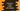# How to create a class in typescript with example## How to create a class in typescript with examples:

Classes are a main component of object oriented programming. A class is a blueprint or prototype with different properties and methods defined by the user. Using this prototype or class, objects are created. We can create objects with different properties and all objects will have the same methods but work differently. This feature was introduced in Typescript from ES6.

In this post, I will give you a quick introduction to class in typescript with examples.

### How to create a class:

class keyword is used to define a class. Inside a class, we can have different fields or properties, constructor and other functions. The constructors are used to intialize the fields in the class.

For example, let’s create a class of Student which can store the name and age of a student.

``````class Student{
name: string;
age: number;

constructor(_name: string, _age: number){
this.name = _name;
this.age = _age;
}
}``````

Here, we created a class with two properties name and age. name is of string type and age is of number type. The constructor is like a function that takes the values and assign them to the properties.

### Create objects for class:

Now, let’s create two objects of the Student class. We will assign two different values for these objects.

``````class Student{
name: string;
age: number;

constructor(_name: string, _age: number){
this.name = _name;
this.age = _age;
}
}

let std1 = new Student('Alex', 20);
let std2 = new Student('Bob', 21);

console.log('std1 name: '+std1.name+', age: '+std1.age);
console.log('std2 name: '+std2.name+', age: '+std2.age);``````

Here,

• We have created two Student objects with different name and age values.
• The last two log statements are printing the values of these two objects.

If you run this program, it will print the below output:

``````std1 name: Alex, age: 20
std2 name: Bob, age: 21``````

As you can see, both objects have different values.

### Accessing methods of a class:

We can also access the methods of a class similarly. For example,

``````class Student{
name: string;
age: number;

constructor(_name: string, _age: number){
this.name = _name;
this.age = _age;
}

printValues(){
console.log('name: '+this.name+', age: '+this.age);
}
}

let std = new Student('Alex', 20);
std.printValues();``````

Here,

• printValues is a method to print the values of the current object. It uses this to access them.
• We have created the object std and calling this method.

It will print the below output:

``name: Alex, age: 20``